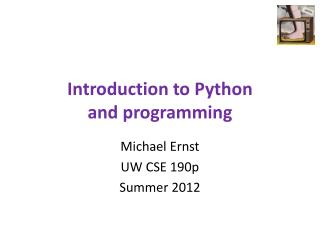DownloadDownload PresentationIntroduction to Python and programming

# Introduction to Python and programming

Download Presentation## Introduction to Python and programming

- - - - - - - - - - - - - - - - - - - - - - - - - - - E N D - - - - - - - - - - - - - - - - - - - - - - - - - - -
##### Presentation Transcript

1. Introduction to Pythonand programming Michael Ernst UW CSE 190p Summer 2012

2. 1. Python is a calculator 2. A variable is a container 4. A program is a recipe 3. Different types cannot be compared

3. 0. Don’t panic! • CSE 190p is for people who have never programmed • (If you have programmed, you don’t belong here.) • You can learn to program in 9 weeks • You will work hard • We will work hard to help you • Ask questions! • This is the best way to learn

4. 1. Python is a calculator

5. You type expressions.Python computes their values. • 5 • 3+4 • 44/2 • 2**3 • 3*4+5*6 • If precedence is unclear, use parentheses • (72 – 32) / 9 * 5

6. An expression is evaluatedfrom the inside out an expression values • (72 – 32) / 9.0 * 5 • (72 – 32) / 9.0 * 5 • (40) / 9.0 * 5 • 40 / 9.0 * 5 • 4.44 * 5 • 22.2 How many expressions are in this Python code?

7. Another evaluation example • (72 – 32) / (9.0 * 5) • (40) / (9.0 * 5) • 40 / (9.0 * 5) • 40 / (1.8) • 40 / 1.8 • 22.2

8. 2. A variable is a container

9. Variables hold values No output from an assignment statement • Recall variables from algebra: • Let x = 2 … • Let y = x … • To assign a variable, use “varname = expression” pi = 3.14 pi avogadro= 6*10**23 avogadro 22 = x # Error! • Not all variable names are permitted

10. Changing existing variables(“re-binding” or “re-assigning”) x = 2 x y = 2 y x = 5 x y

11. Changing existing variables(“re-binding” or “re-assigning”) x = 2 x y = x y x = 5 x y • “=” in an assignment is not a statement or promise of eternal equality • Evaluating an expression gives a new (copy of a) number, rather than changing an existing one

12. How an assignment is executed State of the computer: Printed output: 2 2 3 5 2 3 x: 2 x: 5 y: 2 z: 3 To visualize a program’s execution:http://people.csail.mit.edu/pgbovine/python/tutor.html • Evaluate the right-hand side to a value • Store that value in the variable x = 2 print x y = x print y z = x + 1 print z x = 5 print x print y print z

13. More expressions: Conditionals Numeric operators: +, *, ** Boolean operators: not, and, or Mixed operators: <, >=, == 22 > 4 22 < 4 22 == 4 x = 100 # Assignment, not conditional! 22 = 4 # Error! x >= 5 x >= 100 x >= 200 not True not (x >= 200) 3<4 and 5<6 4<3 or 5<6 temp = 72 water_is_liquid = temp > 32 and temp < 212

14. More expressions: strings A string represents text 'Python' myclass = "CSE 190p" "" Empty string is not the same as an unbound variable Operations: • Length: len(myclass) • Concatenation: "Michael" + 'Ernst' • Containment/searching: '0'in myclass "O" in myclass

15. 3. Different types cannot be compared

16. Types of values • Integers (int): -22, 0, 44 • Arithmetic is exact • Some funny representations: 12345678901L • Real numbers (float, for “floating point”): 2.718, 3.1415 • Arithmetic is approximate, e.g., 6.022*10**23 • Some funny representations: 6.022e+23 • Strings (str): "I love Python", "" • Truth values (bool, for “Boolean”):True, False George Boole

17. Operations behave differentlyon different types 3.0 + 4.0 3 + 4 3 + 4.0 "3" + "4" 3 + "4" # Error 3 + True # Insanity! Moral: Python sometimes tells you when you do something that does not make sense.

18. Operations behave differentlyon different types 15.0 / 4.0 15 / 4 # Insanity! 15.0 / 4 15 / 4.0 Type conversion: float(15) int(15.0) int(15.5) int(“15”) str(15.5) float(15) / 4

19. 4. A program is a recipe

20. What is a program? A program is a sequence of instructions The computer executes one after the other, as if they had been typed to the interpreter Saving as a program is better than re-typing from scratch x = 1 y = 2 x + y print x + y print "The sum of", x, "and", y, "is", x+y

21. Exercise: Convert temperatures • Make a temperature conversion chart:Fahrenheit to Centrigrade, for -40, 0, 32, 68, 98.6, 212, 293, 451Output: -40 -40.0 0 -17.7778 32 0.0 68 20.0 98.6 37.0 212 100.0 293 145.0 451 232.778 • You have created a Python program! • (It doesn’t have to be this tedious, and it won’t be.)

22. 1. Python is a calculator 2. A variable is a container 4. A program is a recipe 3. Different types cannot be compared